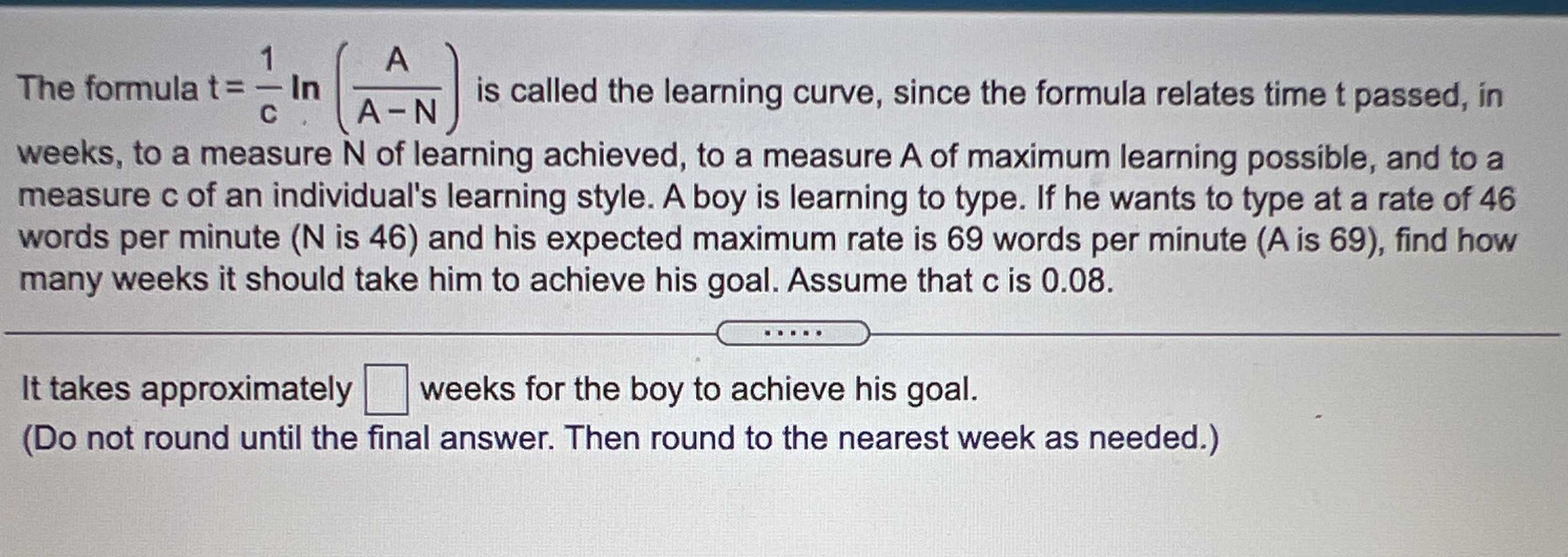### Still have math questions?

Algebra
QuestionThe formula $$t = \frac { 1 } { c } \ln ( \frac { A } { A - N } )$$ is called the learning curve, since the formula relates time t passed, in weeks, to a measure $$N$$ of learning achieved, to a measure $$A$$ of maximum learning possible, and to a measure c of an individual's learning style. A boy is learning to type. If he wants to type at a rate of 46 words per minute ( $$N$$ is 46 ) and his expected maximum rate is 69 words per minute (A is 69 ), find how many weeks it should take him to achieve his goal. Assume that $$c$$ is $$0.08$$ .

It takes approximately $$\square$$ weeks for the boy to achieve his goal.

(Do not round until the final answer. Then round to the nearest week as needed.)